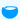Math Calculator

## Hemisphere CalculatorVolume of Hemisphere: [ (2/3)πr³ ] Enter the Radius = Volume of Hemisphere =Curved Surface Area(CSA) of Hemisphere: [ 2πr² ] Enter the Radius = Curved Surface Area(CSA) of Hemisphere =Total Surface Area(TSA) of Hemisphere: [ 3πr² ] Enter the Radius = Total Surface Area(TSA) of Hemisphere =